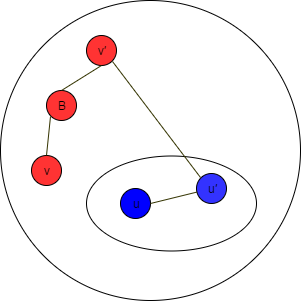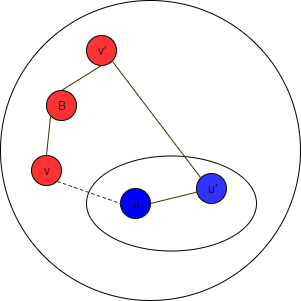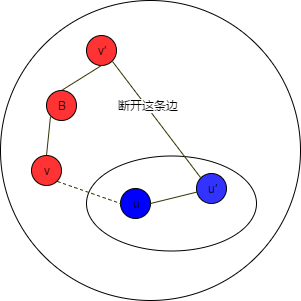### MST性质

MST性质就是最小生成树性质：

```设
连通网G = (V, E)，
U是V的非空真子集，
边(u, v)是 所有一端在U中，另一端在V-U的边 中，代价最小的

在G的所有最小生成树中，一定有一棵包含(u, v)
```

### MST性质的证明• `cost(T′) = cost(T) + cost(u, v) - cost(u′, v′)`
• `cost(u, v) <= cost(u′, v′)`